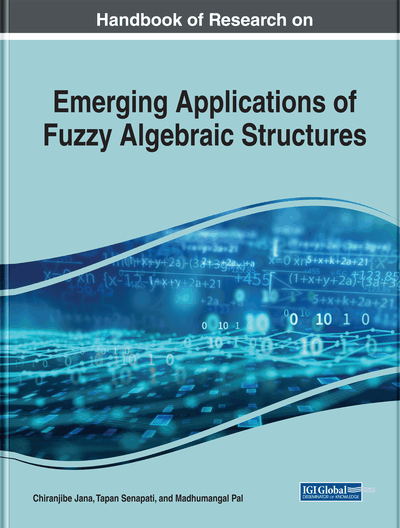Characterizations of Fuzzy Sublattices Based on Fuzzy Point

Chiranjibe Jana (Vidyasagar University, India) and Faruk Karaaslan (Cankiri Karatekin University, Turkey)
DOI: 10.4018/978-1-7998-0190-0.ch007
Available
\$37.50
No Current Special Offers

Abstract

In a lattice 𝔏, the authors used the concept of belongingness and quasi-coincidence of fuzzy point to a fuzzy set, and by this notion, (∈,∈∨q)-fuzzy sublattice, (∈,∈∨q)-fuzzy ideal, cartesian product of (∈,∈∨q)-fuzzy sublattice, (∈,∈∨q)-fuzzy complemented sublattice, and cartesian product of (∈,∈∨q)-fuzzy complemented sublattice are introduced, and their properties are briefly studied. The relationship between fuzzy sublattice and (∈,∈∨q)-fuzzy sublattice, fuzzy ideal and (∈,∈∨q)-fuzzy ideal of L are established. The authors prove that the cartesian product of two (∈,∈∨q)-fuzzy ideals of a lattice is not necessarily a fuzzy ideal of a lattice. The theory of image and inverse image of an (∈,∈∨q)-fuzzy sublattice and (∈,∈∨q)-fuzzy ideal, an (∈,∈∨q)-fuzzy complemented sublattice, and (∈,∈∨q)-fuzzy complemented ideal of 𝔏 on the basis of homomorphism of lattices are also significantly established.
Chapter Preview
Top

1 Introduction

The concept of fuzzy sets proposed by Zadeh in 1965 (Zadeh, 1965). After the initiation of fuzzy sets by Zadeh, there is a huge number of generalization of fuzzy sets in many areas, fuzzification of algebraic structure is one of them. Fuzzy algebraic structures play an important role in mathematics and have a huge scientific applications in theoretical physics, computer sciences, control engineering, information science, coding theory, topological spaces and so on. Then researchers are strongly motivated to review their concepts and results in various areas of abstract algebras in broader frame of fuzzy setting.

Rosenfeld introduced the notion of fuzzy subgroup of a group, base on this fundamental concept many researchers generalizes this idea in many structures (Rosenfeld, 1971), Mordeson and Malik study on fuzzy subring and fuzzy ideal in ring theory (Mordeson, & Malik., 1998). Jun, (Jun, 2005), Jun et al. (Jun, Öztürk, &Muhiuddin., 2016), Meng and Guo (Meng, & Guo., 2005), Ma et al.(Ma, Zhan, & Davvaz., 2008), (Ma, Zhan, & Jun., 2009), (Ma, Zhan, & Jun., 2011) applied fuzzy set theory based on fuzzy points in BCK/BCI-subalgebras and ideals of BCK/BCI -algebras. He et al.(He, Xin, & Zhan., 2014), (He, Xin, & Zhan., 2016) and Zhang and Zhan (Zhang, & Zhan., 2017) have been done lot of work on lattices, Khan et al. (Khan, Davvaz, Yaqoob, Gulistan, & Khalaf., 2015) studied briefly on (∈,∈∨qk) -fuzzy KU-ideals and (∈,∈∨qk)-fuzzy ideals on subtraction algebras, Muhiuddin and Al-roqi (Muhiuddin, & Al-roqi., 2015) focused to the study of subalgebras of BCK/BCI -algebras based on (α,β) -type fuzzy sets, Jana et al. (Jana et al., 2015, 2015a, 2016, 2016a, 2017, 2017a), Bej and Pal (Bej, & Pal., 2015) have done detailed investigation in BCK/BCI -algebras and BG/G-algebras. Bhakat and Das analyzed a new kind of fuzzy subgroup by using the notion of belongings to (∈)and quasi-coincident of a fuzzy point xa to a fuzzy set A in group theory (Bhakat, & Das., 1996a), (Bhakat, & Das., 1996b). Now, we review one of the structures that are most extensively used and discussed in mathematics and its applications are lattices done by Birkhoof one hand which is considered as a relational, ordered structure, on the other hand considered as an algebras (Birkhoff, 2009). Thereafter many researchers generalized lattices by using the fuzzy set theory and developed different fuzzy lattice structures such as Bo and Wangming introduced the concept of fuzzy ideal theory in distributive lattices (Bo, & Wangming., 1990). The definition of 𝔏 -fuzzy sets introduced by Tepavcevic and Trajkovski (Tepavcevic, & Trajkovski., 2001), Seselja et al. introduced the notion of representation of fuzzy lattice and codes by using the 𝔏 -fuzzy lattices (Seselja, & Tepavcevic., 1994), (Seselja, Tepavcevic, & Vojvodic., 1993), Ali and Ray studied product on fuzzy sublattices (Ali, & Ray., 2007), Feng et al. introduced the concept of (λ,γ) -fuzzy sublattice and (λ,γ)-fuzzy subhyperlattice, and described the Cartesian product of (λ,γ)-fuzzy sublattices, and product of subhyperlattice and interval-valued subhyperlattice (Feng, & Zou., 2010), (Feng, Duan, & Zeng., 2010), (Feng, Zeng, Zou, & Zhang., 2010). Recently, Kazanci and Davvaz studied the generalized fuzzy sublattices by means of fuzzy points (Kazancı, & Davvaz., 2010). Ali et al. studied (∈,∈∨q)-fuzzy equivalence relation by using indistinguishability operator (Ali, Feng, & Shabir., 2012). Motivated by the above works and best of our knowledge there is no work available on (∈,∈∨q)-fuzzy sublattice and (∈,∈∨q)-fuzzy ideal of a complemented lattices, of their homomorphism and their Cartesian product of (∈,∈∨q)-fuzzy sublattice of a complemented lattice. For this reason we developed a theoretical study on characterization of fuzzy sublattice and ideals.

Complete Chapter List

Search this Book:
Reset